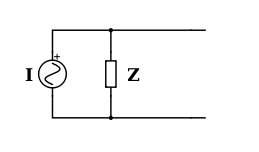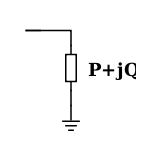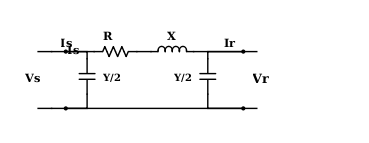# Modelling the Components for a Load Flow Analysis

The Load Flow Analysis is done to determine the flow of real and reactive between different buses in a power system.  It also helps in determining the voltage and current at different locations.

To conduct a Load Flow Analysis, the components in a power system need to be modelled.  The modelling is done by developing equivalent circuits of the components, such as the generator, transmission lines and line capacitances.

The Generator equivalent circuit is shown below.

The Thevenin equivalent circuit is as shown below.This consists of a voltage source and a resistance and an inductance in series with the load.

E = V + IZ

where

Z is the steady stage impedance
V is the voltage and
I is the current

The Norton equivalent circuit

The Norton equivalent circuit consists of a power source and an admittance in parallel.INorton = V/Z

INorton = YVThe load is modelled as a resistance and inductance in a series circuit that is earth

Transmission lines

Transmission lines are modelled as

Short transmission lines (less than 80 km)

Short lines that are less than 80 kms long are modelled as a resistance and reactance in series with the load.  The line capacitances are neglected.

Medium (80 to 250 km)

Medium lines are modelled as a resistance and reactance in series.  The admittance is in parallel in two sections.Long lines ( 250 km and above)

Long lines are also modelled as a resistance and reactance in series.  The admittance is in parallel in two sections.